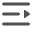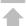# Linux 环境推荐配置

## 调整 ulimit

1. 在配置文件 /etc/security/limits.conf 中设置：

``` #<domain>      <type>    <item>        <value>
*              soft        core           0
*              soft        data           unlimited
*              soft       fsize           unlimited
*              soft          as           unlimited
*              soft      nofile           65535```

参数说明

core：数据库出现故障时产生 core 文件用于故障诊断，生产系统建议关闭；

data：数据库进程所允许分配的数据内存大小；

fsize：数据库进程所允许寻址的文件大小；

as：数据库进程所允许最大虚拟内存寻址空间限制；

nofile：数据库进程所允许打开的最大文件数；

2. 在配置文件 `/etc/security/limits.d/90-nproc.conf` 中设置：

``` #<domain>      <type>    <item>     <value>
*              soft       nproc      unlimited
*              hard       nproc      unlimited```

参数说明：

nproc：数据库所允许的最大线程数限制；

Note:

• 每台作为数据库服务器的机器都需要配置
• 更改配置后需重新登录使得配置生效

## 调整内核参数

1. 使用下列命令输出当前 vm 配置，并将其归档保存：

``` \$ cat /proc/sys/vm/swappiness
\$ cat /proc/sys/vm/dirty_ratio
\$ cat /proc/sys/vm/dirty_background_ratio
\$ cat /proc/sys/vm/dirty_expire_centisecs
\$ cat /proc/sys/vm/vfs_cache_pressure
\$ cat /proc/sys/vm/min_free_kbytes
\$ cat /proc/sys/vm/overcommit_memory
\$ cat /proc/sys/vm/overcommit_ratio```
2. 添加下列参数至 `/etc/sysctl.conf` 文件调整内核参数：

``` vm.swappiness = 0
vm.dirty_ratio = 100
vm.dirty_background_ratio = 40
vm.dirty_expire_centisecs = 3000
vm.vfs_cache_pressure = 200
vm.min_free_kbytes = < 物理内存大小的 8%，单位 KB (kbytes)。最大不超过 1GB (即 1048576KB)。>
vm.overcommit_memory = 2
vm.overcommit_ratio = 85```

Note:
当数据库可用物理内存不足 8GB 时不需使用 vm.swappiness = 0；上述 dirty 类参数只是建议值，具体系统设置时请按原则（控制系统的 flush 进程只采用脏页超时机制刷新脏页，而不采用脏页比例超支刷新脏页）进行设置。
如果是ssd盘，建议 vm.dirty_expire_centisecs = 1000。

3. 执行如下命令，使配置生效：

` \$ /sbin/sysctl -p  `
4. ssd盘建议调整预读大小和块层读写请求数：

• 确定块设备

当前环境存在 `sd[a-l]` 12 块块设备

```\$ ls /sys/block/
sda  sdb  sdc  sdd  sde  sdf  sdg  sdh  sdi  sdj  sdk  sdl```
• 确定磁盘是否是 SSD

建议 fio 测试一下块设备的随机读写的 IOPS (我们希望采用的 SSD 盘有上万的IOPS) 来确定，或者咨询系统管理员

```      \$ fio -filename=/data/disk_ssd2/test -direct=1 -iodepth 1 -thread -rw=randrw -rwmixread=70 -ioengine=psync -bs=4k -size=500G -numjobs=50 -runtime=180 -group_reporting -name=ranrw_70read_4k_local
ranrw_70read_4k_local: (g=0): rw=randrw, bs=(R) 4096B-4096B, (W) 4096B-4096B, (T) 4096B-4096B, ioengine=psync, iodepth=1
...
fio-3.7
ranrw_70read_4k_local: Laying out IO file (1 file / 512000MiB)
ranrw_70read_4k_local: Laying out IO file (1 file / 512000MiB)
Jobs: 50 (f=50): [m(50)][100.0%][r=103MiB/s,w=44.0MiB/s][r=26.4k,w=11.5k IOPS][eta 00m:00s]
ranrw_70read_4k_local: (groupid=0, jobs=50): err= 0: pid=1322291: Thu Oct 24 12:01:56 2019
clat (usec): min=33, max=6654, avg=1386.15, stdev=1112.59
lat (usec): min=33, max=6654, avg=1386.30, stdev=1112.59
clat percentiles (usec):
|  1.00th=[  135],  5.00th=[  149], 10.00th=[  159], 20.00th=[  178],
| 30.00th=[  212], 40.00th=[  469], 50.00th=[ 1663], 60.00th=[ 1926],
| 70.00th=[ 2147], 80.00th=[ 2474], 90.00th=[ 2802], 95.00th=[ 3032],
| 99.00th=[ 3752], 99.50th=[ 4228], 99.90th=[ 4817], 99.95th=[ 5014],
| 99.99th=[ 5276]
bw (  KiB/s): min= 1776, max= 2632, per=2.00%, avg=2138.01, stdev=101.57, samples=17964
iops        : min=  444, max=  658, avg=534.47, stdev=25.39, samples=17964
# 这里可以看到当前场景下，write 的IOPS 为 11500
write: IOPS=11.5k, BW=44.8MiB/s (46.0MB/s)(8064MiB/180004msec)
clat (usec): min=29, max=5153, avg=1122.50, stdev=1030.29
lat (usec): min=29, max=5153, avg=1122.73, stdev=1030.29
clat percentiles (usec):
|  1.00th=[   38],  5.00th=[   45], 10.00th=[   48], 20.00th=[   55],
| 30.00th=[   61], 40.00th=[   77], 50.00th=[ 1467], 60.00th=[ 1762],
| 70.00th=[ 1958], 80.00th=[ 2180], 90.00th=[ 2442], 95.00th=[ 2606],
| 99.00th=[ 2835], 99.50th=[ 2933], 99.90th=[ 3097], 99.95th=[ 3163],
| 99.99th=[ 3326]
bw (  KiB/s): min=  528, max= 1368, per=2.00%, avg=917.29, stdev=96.92, samples=17964
iops        : min=  132, max=  342, avg=229.30, stdev=24.23, samples=17964
lat (usec)   : 50=3.90%, 100=9.07%, 250=25.19%, 500=4.08%, 750=1.97%
lat (usec)   : 1000=1.10%
lat (msec)   : 2=20.66%, 4=33.52%, 10=0.51%
cpu          : usr=0.31%, sys=1.88%, ctx=13575553, majf=1, minf=5
IO depths    : 1=100.0%, 2=0.0%, 4=0.0%, 8=0.0%, 16=0.0%, 32=0.0%, >=64=0.0%
submit    : 0=0.0%, 4=100.0%, 8=0.0%, 16=0.0%, 32=0.0%, 64=0.0%, >=64=0.0%
complete  : 0=0.0%, 4=100.0%, 8=0.0%, 16=0.0%, 32=0.0%, 64=0.0%, >=64=0.0%
issued rwts: total=4811226,2064291,0,0 short=0,0,0,0 dropped=0,0,0,0
latency   : target=0, window=0, percentile=100.00%, depth=1

Run status group 0 (all jobs):
READ: bw=104MiB/s (109MB/s), 104MiB/s-104MiB/s (109MB/s-109MB/s), io=18.4GiB (19.7GB), run=180004-180004msec
WRITE: bw=44.8MiB/s (46.0MB/s), 44.8MiB/s-44.8MiB/s (46.0MB/s-46.0MB/s), io=8064MiB (8455MB), run=180004-180004msec

sdh: ios=4806033/2062158, merge=0/35, ticks=1475846/96883, in_queue=1572092, util=99.66%```
• 调整 SSD 盘预读大小和块层读写请求数，根据前面确定的 SSD 来调整
```\$ vi /etc/profile
# 修改第一块 SSD 配置， 这里的 sdg 是前面确定的 SSD 设备
echo 256 >/sys/block/sdg//queue/nr_requests

# 修改第二块 SSD 配置， 这里的 sdh 是前面确定的 SSD 设备
echo 256 >/sys/block/sdh//queue/nr_requests
...```

Note:
上面的设备号 (如: sdg) 需要先确定，并且要确定是不是 SSD 类型。

## 关闭 transparent_hugepage

1. 编辑 `/etc/rc.local`，在第一行 “#!/bin/sh” 的下一行添加如下两行内容：

``` echo never > /sys/kernel/mm/transparent_hugepage/enabled
echo never > /sys/kernel/mm/transparent_hugepage/defrag```
2. 执行如下命令，使配置生效：

` \$ source /etc/rc.local`
3. 检查是否成功关闭transparent_hugepage。分别执行如下两条命令：

``` \$ # 若关闭成功，显示效果如下：
\$ cat /sys/kernel/mm/transparent_hugepage/enabled
\$ cat /sys/kernel/mm/transparent_hugepage/defrag

\$ # 若关闭失败，显示效果如下：
\$ cat /sys/kernel/mm/transparent_hugepage/enabled
\$ cat /sys/kernel/mm/transparent_hugepage/defrag

## NUMA的影响

### 关闭NUMA

1.关闭NUMA的方案:

• 方案一：建议使用该方案，开机按快捷键进入BIOS设置界面，关闭NUMA，保存设置并重启，再执行后续步骤验证是否成功关闭NUMA。不同品牌的主板或服务器，具体操作略有差异，此处不作详细介绍。

• 方案二：修改grub的配置文件，关闭NUMA：

• 对CentOS6.4的grub配置文件修改

以root权限编辑 /etc/grub.conf ，找到"kernel"引导行，该行类似如下（不同的版本内容略有差异，但开头有“kernel /vmlinuz-”）：

`kernel /vmlinuz-2.6.32-358.el6.x86_64 ro root=/dev/mapper/vg_centos64001-lv_root rd_NO_LUKS rd_LVM_LV=vg_centos64001/lv_root rd_NO_MD rd_LVM_LV=vg_centos64001/lv_swap crashkernel=128M LANG=zh_CN.UTF-8  KEYBOARDTYPE=pc KEYTABLE=us rd_NO_DM rhgb quiet`

在kernel行的末尾，空格再添加 “numa=off” ，如果有多个kernel行，则每个kernel行都要添加。

• 对Ubuntu12.04的grub文件修改

以root权限编辑 /boot/grub/grub.cfg ，找到"linux"引导行，该行类似如下（不同版本内容略有差异，但开头有“linux /boot/vmlinuz-”）：

`linux   /boot/vmlinuz-3.2.0-31-generic root=UUID=92191cd8-3690-4cd4-9f42-95d392c9d828 ro`

在Linux引导行的末尾，空格再添加 “numa=off” ，如果有多个Linux引导行，则每个Linux引导行都要添加。

• 修改后保存，再重启系统，再执行后续步骤验证是否成功关闭NUMA。

2.验证NUMA是否成功关闭，shell执行如下命令：

`\$ numastat`

Note:

## 数据库目录结构收起